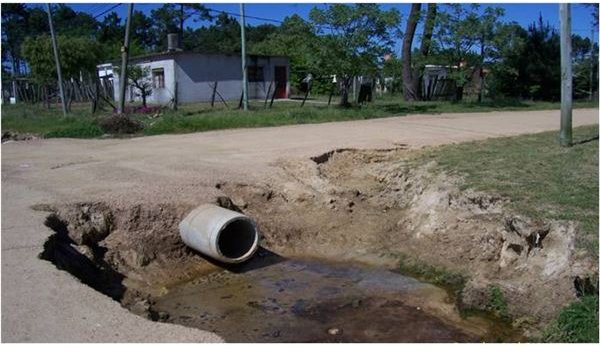How To Calculate Drainage Systems for Secondary Roads

Selecting a Method

We first know that we will probably have to put drains in every low point of a secondary road. By secondary road, we usually mean a rural road built and maintained by a local authority.

To calculate the maximum flow expected to cross from one side of the road to the other, we will first have to set the point where we will put the drain (in a map), then draw the path from where water comes (stream), and determine the basin from contour lines.

After that we have to look for this information in the map:

• L (km): Stream's course length
• dH (m): Elevation difference between the upper point of the stream and the placement of the drain
• A (Há): Basin's area

In this way we can calculate the stream's slope as: S (%)=dH/(L*10), and the time of concentration with the Kirpich equation:

tc(hs)=(0.4).L0.77.S-0.385

This data allows us to choose the method we have to calculate the peak flow for a determined storm's return period, so we procceed in this way:

• If tc < 20 minutes: calculate peak flow with Rational Method
• If tc > 20 minutes and A > 400 Há.: calculate peak flow with SCS Method
• If tc > 20 minutes and A < 400 Há.: calculate peak flow with Rational and SCS Methods, then choose the higher flow value.

Rational Method

We first define the storm's return period: Tr. To have an idea, generally a two-year storm is used in secondary roads, but this is a parameter you will manage in the order of the road importance and the risks derived from the drain collapse.

We need the information about the sample precipitation for the place we will put the drain: P3,10 (mm). This is a precipitation of 3 hours duration and 10 years return period and it is drawn in a precpitation lines map.

With this data we can calculate the correction coefficient for the period return as: CT = 0.5786 – 0.4312. Log10(Ln(Tr/(Tr-1))), and the correction coefficient for the storm duration:

• If tc < 3hs: CD = 0.6208.tc/((tc+0.0137)0.5639)
• If tc > 3hs: CD = 1.0287.tc/((tc+1.0293)0.8083)

Then we calculate precipitation as: P(mm) = P3,10 . CT. CD, and then intensity: i (mm/h) = P/tc

The runoff coefficient c depends on the return period and ground cover and it's adimensional. Now we can calculate the flow peak as:

Q (m3/s) = c. i. A/360

SCS Method

This is the Soil Conservation Service method to calculate peak flow for certain basins. The information we have to collect to work with this method doesn't vary enough from the Rational Method although the theory is hardly different at all. Moreover beside the return period, we have to specify the Hydrologic Soil Type and the Curve Number after we know the soil uses. We find this data in specific charts.

Using this information we can draw the triangular hydrogram: Q = f (t). This hydrogram represents the drain produced in a 1 cm area of a particular rain on the basin. Then we draw a new hydrogram summing the out of phase triangular hydrograms during the storm duration. The peak flow sought is the maximum flow value found in this hydrogram.

Calculating Drains

After we have the peak flow value for the selected return period, we can use the HLW software for example, and calculate the drainage area needed. Remember you must limit the water velocity, which must be less than 5 m/s for concrete. Even though, this is a vecinal road, so the bottom and slopes of the stream are made up of earth. This will reduce the acceptable limit of velocity to avoid erosion.

Once you calculate the necessary area to let the water cross from one side to the other of the road, you can choose the number and type of drains to this low point, considering commercial drains.